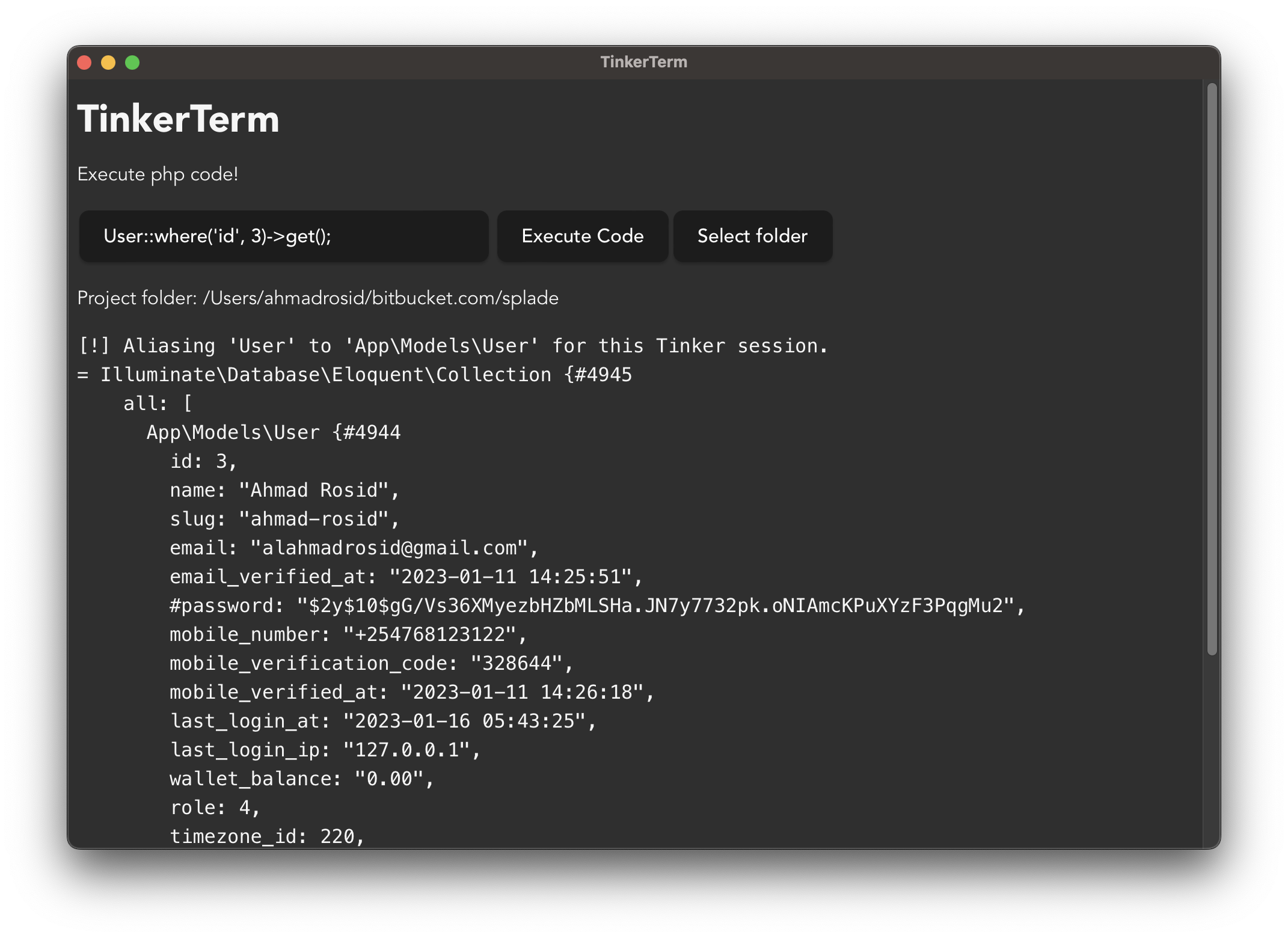# How to run command with custom stdin and stdout in Rust?self.__wrap_balancer=(t,e,n)=>{n=n||document.querySelector(`[data-br="\${t}"]`);let o=n.parentElement,r=E=>n.style.maxWidth=E+"px";n.style.maxWidth="";let i=o.clientWidth,s=o.clientHeight,c=i/2,u=i,d;if(i){for(;c+1<u;)d=~~((c+u)/2),r(d),o.clientHeight==s?u=d:c=d;r(u*e+i*(1-e))}};self.__wrap_balancer(":R2sm:",1)

So, this week I am working on some little side project to run php code for laravel project with `php artisan tinker`.So the problem with this command it's does not support passing some code via input params. And basically this command was interactive repl.

My idea to get this working is to controll the `stdin` and `stdout` of the command. Luckily rust make it easier for us to implement this kind of behaviour.

Let's have a function that will call the command. We need to set where is the directory will be when we execute the command. So for this we can use `PathBuf`.

``````fn tinker_run(code: &str, path: String) -> String {
let path = PathBuf::from(path);
tinker::run(code.to_string(), path)
}
``````

And now here's the complete.

``````use std::io::Write;
use std::path::PathBuf;
use std::process::{Command, Stdio};

pub fn run(code: String, path: PathBuf) -> String {
let mut child = Command::new("php")
.stdin(Stdio::piped())
.stderr(Stdio::piped())
.stdout(Stdio::piped())
.current_dir(path)
.arg("artisan")
.arg("tinker")
.spawn()
.expect("Failed to spawn child process");

let mut stdin = child.stdin.take().expect("Failed to open stdin");
stdin
.write_all(code.as_bytes())
.expect("Failed to write to stdin");
});

let output = child.wait_with_output().expect("Failed to read stdout");
String::from_utf8_lossy(&output.stdout).to_string()
}
``````

Here is the explanation :

• The `stdin(Stdio::piped())`, `stderr(Stdio::piped())`, and `stdout(Stdio::piped())` methods are used to set up pipes for the standard input, standard error, and standard output of the child process, respectively.
• The `current_dir(path)` method is used to set the current working directory of the child process to the path provided as a function argument.
• The `spawn()` method is used to start the child process and returns a Child struct. The expect("Failed to spawn child process") is used to handle any errors that might occur when starting the child process.
• The `stdin` field of the `Child` struct is taken and used to write the code string passed to the function as argument.
• `std::thread::spawn` is used to create a new thread which will execute the closure passed to it.
• Finally, the `wait_with_output()` method is used to wait for the child process to exit and collect its output. The output is converted to a string and returned as the output of the function.

Built with Next.js, Tailwind and Vercel